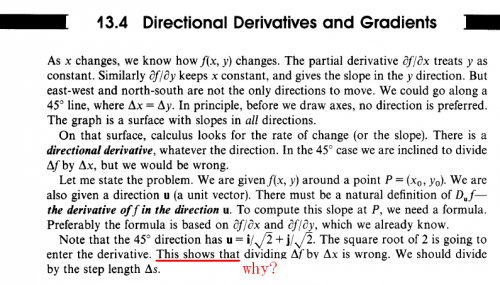# bingliantech

Members

7

## Posts posted by bingliantech

1. ### one question about the substitution in multiple integrals

I know the Jacobian of the coordinate transformation measures how much the transformation is expanding or contracting the area around a point in G as G is transformed into R.

My question is why we need the Jacobian in the transformation,the deltaA which represent small area go into almost zero in the integral,and the deltaS which represnt the transformed small area also go into zero,why we need the Jacobian to measure the multiple.

2. ### understanding about directional derivative

OK,no problem,i considered M which is {y1,y2,y3..yp...}⊂M as co-domain in the past,i can understand your steps and i think i can understand partial derivative.

3. ### understanding about directional derivative

In particular he has mixed the vector based approach with the traditional geometric one, omitting important parts of both.

We can discuss this further if you wish.

Are you familiar with the vector dot product?

Yes,i found Strang's linear algebra is very good and it's the reason why i want reading his calculus carefully.

I generally read his linear algebra several days ago so i think i may have the basic understanding of vector dot product.

Could you tell me the omiting parts ?I can understand f should be devided by s,it's not difiicult to be understood,i just be puzzled with his reason or his explanation.

to imatfaal

It's a good idea to watch the video of lecture,but i don't listen english often,maybe i can make a try.

Thank you!

4. ### understanding about directional derivative

Thank you very much, just as you said, the authour may want to say if we divide f by x , we will get root 2* f/s for limit,only f/s is right, i am not very customed with the expression in the last paragragh.

Thanks a lot for studiot and imatfaal's help and these paragraphs come from strang's "calculus".

5. ### understanding about directional derivative

Thank you for your reply, i can understand what you have said as i can understand the first three paragraghs in the picture,but the last paragraph puzzle me,i can't get why the square root of 2 going to enter shows that dividing delta f by delta x is wrong.

6. ### understanding about directional derivative

I am learing directional derivative and one context in the book puzzle me, i know delta f should be divided by

delta s not delta x when we use directional derivative,but i really can't understand the explain in the book.

Anyone can help me ?7. ### how to delete the topic？

how to delete the topic？

×